# Falsifying the log growth model of Bitcoin value

## ABSTRACT

This article explores if there is a relationship of time to Bitcoin price. The proposed log-log model [1, 2 & 3] is tested for statistical validity against the least squares assumptions, for stationarity in each variable and for potential spurious relationships using the Engle-Granger approach for cointegration. All but one of these tests are able to reject the hypothesis that time is an important predictor for Bitcoin price.

## INTRODUCTION

A log price ~ log time (aka log growth) model has been proposed by various source [1, 2 & 3] as explaining a large proportion of bitcoin price movements and consequently has been put forward as a mechanism to estimate future bitcoin prices.

Scientific method is difficult for most to comprehend. It is counterintuitive. It can lead to conclusions that do not reflect personal beliefs. It takes a foundation in the method to understand this basic fundamental concept: it is ok to be wrong.

According to the great modern scientific philosopher Karl Popper, testing a hypothesis for an incorrect outcome is the only reliable way to add weight to the argument that it is correct. If rigorous and repeated tests cannot show that a hypothesis is incorrect, then with each test the hypothesis assumes a higher likelihood of being correct. This concept is called Falsifiability. This article aims to falsify the log growth model of Bitcoin value, as basically defined in [1, 2 & 3]

## NOTES

• All analysis was performed using Stata 14.
• This is not financial advice.

## DEFINING THE PROBLEM

To falsify a hypothesis, first we must state what it is:

Null Hypotheses (H0): The price of Bitcoin is a function of the number of days Bitcoin has existed

Alternative Hypotheses (H1): The price of Bitcoin is not a function of the number of days Bitcoin has existed

The authors of [1, 2 & 3] chose to test H0 by fitting an Ordinary Least Squares (OLS) regression on the natural log of the price of Bitcoin and the natural log of the number of days Bitcoin has existed. There was no accompanying diagnostics nor any identified reasoning for the log transformation in both variables. The model did not take into account the possibility of a spurious relationship due to non-stationarity, nor any possibility of interaction or other confounding factors.

## APPROACH

In this article, we will explore that model and run it through the normal regression diagnostics and determine if the log transformation was necessary or appropriate (or both) and explore possible confounding variables, interactions, and sensitivity to confounding.

Another issue that will be explored is that of non-stationarity. Stationarity is an assumption of most statistical models. It is the concept that through time, there is no trend in any moments, for example, if there is no trend in the mean (or variance) with respect to time.

Consequent to the stationarity analysis, we will explore the possibility of cointegration.

## NOTATION

Medium is relatively limited for mathematical notation. The usual notation for an estimate of a statistical parameter is to place a hat on top. Instead, we define the estimate of a term as []. e.g. the estimate of β = [β]. If we are representing a 2x2 matrix, we will do so like this [r1c1, r1c2 \ r2c1, r2c2] etc. Subscripted items are superseded by @ — eg for the 10th position in a vector X we would normally subscript X with 10. We will instead write X@10.

## ORDINARY LEAST SQUARES

Ordinary least squares regression is a way to estimate a linear relationship between two or more variables.

First, let us define a linear model as some function of X that equals Y with some error.

Y = βX+ε

where Y is the dependent variable, X is the independent variable, ε is the error term and β is the multiplier of X. The goal of OLS is to estimate β such that ε is minimised.

In order for [β] to be a reliable estimate, some basic assumptions (known as the Gauss-Markov assumptions ) must be met:

1. There is a linear relationship between the dependent and independent variables
2. The errors are homoscedastic (that is — they have a constant variance)
3. The error is normally distributed with a mean of zero
4. There is no autocorrelation in the error (that is — the errors aren’t correlated with the lag of the errors)

## LINEARITY

We begin by taking a look at the non-transformed to scatter plot of price v days (data from coinmetrics).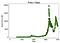Figure 1 — Price v Days. The data are spread across too wide of a range ascertain a linearity visually.

In figure 1, we encounter a good reason to take the log of the price — the span is much too broad. Taking the log of price (but not days) and re-plotting gives us a familiar log looking pattern (figure 2)

Taking the log of the days and again plotting gives us the obvious linear pattern identified by the authors of [1, 2 & 3] in Figure 3.

This confirms the choice of log-log — the only transformations that really show a good linear relationship.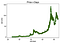Figure 4 — square root transformations aren’t much better than the untransformed series

Thus the preliminary analysis cannot reject H0.

The log-log fitted regression is given in figure 5 below, where [β] = 5.8

Using the model, we can now estimate the residuals [ε] and fitted values [Y] and test the other assumptions.

## HOMOSCEDASTICITY

If the assumption of constant variance in the error term (i.e. homoscedasticity) were to be true, then the error term would vary randomly around 0 for each value in the predicted values. The RVF plot (figure 6) is, therefore, a simple yet effective graphical way to investigate the accuracy of this assumption. In figure 6, we see there is a massive pattern, rather than a random scattering, indicating a non-constant variance in the error term (i.e. heteroscedasticity).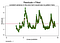Figure 6a — RVF plot. A pattern here indicates there is an issue.

Heteroscedasticity like this causes the estimates of the coefficients [β] to have a larger variance and thus be less precise and leads to p-values that are more significant than they should be, because the OLS procedure does not detect the increased variance. Therefore when we then calculate t-values and F values we use an underestimation of the variance, leading to higher significance. This also has an effect on the 95% confidence interval about [β], which is itself a function of the variance (via the standard error).

The Breusch-Godfrey [6 & 7] statistic for autocorrelation was also significant, further providing evidence of this problem.

At this stage, it would normally be the point at which we would stop and respecify the model. However, given we know the effect of these issues, it will be relatively safe to continue with the regression understanding that these problems exist. There are ways we can deal with (mild forms of) these issues — bootstrapping or using a robust estimator for the variance for example.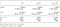Figure 7 — The impact of the heteroscedasticity is shown in the various estimations

As can be seen in figure 7, whilst there is a small increase in the variance (see the broadened confidence interval), for the most part, the heteroscedasticity present isn’t really having too much of a detrimental effect.

## NORMALITY IN ERROR

The assumption that the error term is normally distributed with a mean of zero is a less important assumption to meet than linearity or homoscedasticity. Non-normality, but non-skewed residuals are going to have the effect of making the confidence intervals too optimistic. If the residuals are skewed then you might end up with a little bias. As we can see from figures 8 and 9, the residuals are heavily skewed. A Shapiro-Wilk test for normality gives us a p-value of 0. They do not fit the normal curve enough for the confidence intervals to be unaffected.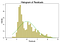Figure 8 — histogram of the error term with a normal distribution (green) overlaid. The error term should be normal, but it isn’t.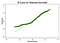Figure 9 — normal quantiles plot of the error term. The closer the dots are to the line the better the normal fit.

# Leverage

Leverage is the concept that not all data points in the regression contribute equally to the estimation of the coefficients. Some points with high leverage could significantly alter the coefficient depending on if they are present or not. In figure 10, we can see quite clearly that there are too many to count concerning points (above the mean residual and above the mean leverage).

## OLS SUMMARY

Basic diagnostics indicate a violations of essentially all of the Gauss-Markov assumptions except for linearity. This is relatively strong evidence to reject H0.

## STATIONARITY

A stationary process is said to be Order 0 integrated (eg I(0)). A non-stationary process is I(1) or more. Integration in this context is more like a poor-mans integration — it is the sum of the lagged difference. I(1) means that if we subtract the first lag from each value in the series, we will have an I(0) process. It is relatively well known that regression on non-stationary time-series can lead to the identification of spurious relationships.

In figures 12 & 13 below, we can see that we cannot reject the null hypothesis of the Augmented Dickey Fuller (ADF) test. The null hypothesis of the ADF test is that the data are non-stationary. This means we cannot say that the data are stationary.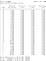Figures 11 & 12 — GLS Augmented Dickey Fuller tests for unit root on log price and log days.

The Kwiatkowski-Phillips-Schmidt-Shin test is a complimentary test for stationarity to the ADF tests. This test (KPSS) has the null hypothesis that the data are stationary. As we can see in figures 13 & 14, we can reject stationarity for most lags in both variables.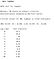Figures 13 & 14 — KPSS test against the null of stationarity

These tests prove that these two series beyond any doubt are non-stationary. This is a bit of a problem. If the series isn’t at least trend stationary then OLS could be misguided into identifying a spurious relationship. One thing we could do is take the log-daily difference of each variable and rebuild our OLS. However; thanks to this issue being rather common in econometric series, there is a much more robust framework available to us — something called cointegration.

## COINTEGRATION

Cointegration is a way to deal with a pair (or more) of I(1) processes and determine if there is a relationship and what that relationship is. To understand cointegration we give a simplified example of a drunk and her dog . Imagine a drunk walking her dog home on a leash. The drunk is walking all over the place, unpredictably. The dog walks pretty randomly as well: sniffing trees, barking, chasing scratching — just generally being a mutt. However, the dog’s overall direction will be within the length of the leash of the drunk. We could estimate that for any point on the drunks walk home, the dog will be within leash length of the drunk (sure it might be on one side or another, but the dog will be within leash length). This bad simplification is a rough metaphor of cointegration — the dog and the owner are moving together.

Contrast this to correlation — Let’s say a stray dog follows the drunk’s mangy mongrel for 95% of the way home and then runs off to chase a car to the other side of town. There would be a very strong correlation between the path of the stray and the drunk (literally R²: 95%), however much like the many one night stands that the drunk has had — that relationship didn’t mean anything — it can’t be used to predict where the drunk will be as whilst for some part of the trip it is true, for some parts it is wildly inaccurate.

In order to find the drunk, first, we will see what lag-order specification our model should use.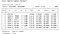Figure 15 — Lag order specification. Minimum AIC is used to determine.

We identify here the most appropriate lag-order to investigate via the selection of the minimum AIC is an order of 6.

Next, we need to identify if there is a cointegrating relationship. The simple Engle-Granger framework [8, 9, 10] makes this relatively easy. If the test statistic is more negative than the critical values, then there is a cointegrating relationship.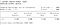Figure 16 — the test statistic is nowhere close to being less than any of the critical values

The results in figure 16 gives us no evidence to say there is a cointegrating equation between log price and log days.

## LIMITATIONS

In this study, we did not account for any confounding variables. Given the evidence above it is very unlikely that any confounders would have a significant impact to our conclusion — we can reject H0. We can say “there is no relationship between log days and log Bitcoin price”. If that were the case, then there would be a cointegrated relationship.

## CONCLUSION

In light of the violations of all bar one of the Gauss Markov assumptions for a valid linear regression and that there is no detectable cointegration, and that both variables are non-stationary, there is sufficient evidence to reject H0, therefore there is not a valid linear relationship between log price and log days, and as such cannot be used to reliably predict out of sample estimates of price.

## CITATIONS

 Davidson, R., and J. G. MacKinnon. 1993. Estimation and Inference in Econometrics. New York: Oxford University
Press.

 Durbin, J., and G. S. Watson. 1950. Testing for serial correlation in least squares regression. I. Biometrika 37:
409–428.

 Engle, R.F. and Granger, C.W.J. 1987. Co-integration and Error Correction: Representation, Estimation and Testing. Econometrica, Vol. 55, pp. 251–276.

 MacKinnon, James G. 1990, 2010. Critical Values for Cointegration Tests. Queen’s Economics Department Working Paper No. 1227, Queen’s University, Kingston, Ontario, Canada. Available at http://ideas.repec.org/p/qed/wpaper/1227.html.

 Schaffer, M.E. 2010. egranger: Engle-Granger (EG) and Augmented Engle-Granger (AEG) cointegration tests and 2-step ECM estimation. http://ideas.repec.org/c/boc/bocode/s457210.html